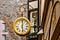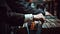# .css-df1pn7{display:block;width:16rem;}# Top 10 Utilities You May Need for Your TypeScript Projects

Deepak Vishwakarma
·Oct 12, 2020·

Subscribe to my newsletter and never miss my upcoming articlesPhoto by Jeff Sheldon on Unsplash

JS|TS By Example

# Top 10 Utilities you may need for your TypeScript Projects

You work on multiple projects. Most of the time you repeat your self while writing common util functions. Here are some of the most useful utilities that you can use for your TypeScript or JavaScript

1. compact: Compact is normally used to remove the falsy value from an Array. There are multiple variations of it.

Simple Variant: filter out falsy values (`false`, `null`, `0`, `""`, `undefined`, and `NaN`).

``````// How to use it
compact([0, 1, false, 2, "", 3, "a", Number("e") * 23, NaN, "s", 34]); _// [ 1, 2, 3, 'a', 's', 34 ]_
``````

Functional and Advanced Variation: You can also take a function as a parameter to define the behavior of the filter.

``````// Code:
type Predicate<T> = (val: T) => boolean;

const compact = <T = any>(fn: Predicate<T>, arr: T[] = []) => arr.filter(fn);
const removeFalsy = curry(compact, 2)(Boolean);
const removeNull = curry(compact, 2)((v: any) => v !== null);

// How to use it
removeFalsy([0, false, "", 3, "a", Number("e") * 23, NaN, null])
// [ 3, 'a']

removeNull([0, false, "", 3, "a", Number("e") * 23, NaN, null]);
// [ 0, false, '', 3, 'a', NaN, NaN, 's' ]
``````

If you noticed, I am using function curry to make compact more functional, and reusable. We will learn curry later in this tutorial.

2. contains The other useful function, I used in most of the projects. After ES 2015, JavaScript does have includes. However, most of the time you end-up error like toLowerCase of undefined is not function. You have to handle boundary cases.

``````// Code:
const contains = (s1: string, s2: string = "") =>
s1.toLowerCase().indexOf(s2.toLowerCase()) !== -1

// How to use it
contains("Text1", "Ext"); _// true_ contains("Text1", "et"); _// false_
``````

Above util is case insensitive in nature. You can update according to your need.

3. curry: If you love functional programming. You can’t skip the curry method in your toolbox. curry is one of the most basic needs of functional programming. However, Defining curry function is TypeScript could be a challenge. Bellow example I defined based on my knowledge. You can find a better variant of it online.

Concept of curry: https://en.wikipedia.org/wiki/Currying

``````// Code:
const curry = (fn: Function, arity = fn.length, ...args: any[]): any => arity <= args.length ? fn(...args) : curry.bind(null, fn, arity, ...args);

// How to use it

const removeFalsy = curry(compact, 2)(Boolean);
const removeNull = curry(compact, 2)((v: any) => v !== null);
``````Photo by Alexander Schimmeck on Unsplash

4. delay: delay is very useful in testing some asynchronous methods. This helps to put some virtual delay to mimic the network call or any async side-effect.

Simple Variant:

``````// Code
const delay = (fn: Function, wait: number, ...args: any[]) =>   setTimeout(fn, wait, ...args);

// How to use it
delay(
function (text) {
console.log(text);
},
1000,
"later"
);
_// Logs 'later' after one second._
``````

Promise Variant: If you needed you can create a Promise variant of it. Just wrap delay in promise.

``````// Code
const delayedPromise = (wait: number = 300, ...args: any[]) =>
new Promise((resolve) => {
delay(resolve, wait, ...args);
});
// How to use it
(async function () {
await delayedPromise();
console.log("Print after delay");
})();
// Print after delay
``````

5. unique: This is used to get the unique values in a given array. This could also have multiple variants. The simplest one uses Set to push values and collect unique value.

``````// Code
const unique = (arr: any[]) => [...new Set(arr)];

// How to use it
unique([1, 2, 2, 3, 4, 4, 5]); // [1, 2, 3, 4, 5]
``````

You can also pass a function to define the uniqueness of the value.

``````// Code
const uniqueBy = (fn: Predicate, arr: any[]) =>
arr.reduce((acc, v) => {
if (!acc.some((x: any) => fn(v, x))) acc.push(v);
return acc;
}, []);

// How to use it
uniqueBy(
(a: any, b: any) => a.id == b.id,
[
{ id: 0, value: "a" },
{ id: 1, value: "b" },
{ id: 2, value: "c" },
{ id: 1, value: "d" },
{ id: 0, value: "e" },
],
);
// [ { id: 0, value: 'a' }, { id: 1, value: 'b' }, { id: 2, value: 'c' } ]
``````

6. sum: Sum is one of the simplest but useful method you may want in your util library. You can use Array.reduce to sum the value.

``````// Code
const sum = (...arr: number[]) => [...arr].reduce((acc, val) => acc + val, 0);

// How to use it
sum(1, 2, 3, 4); // 10
sum(...[1, 2, 3, 4]); // 10
``````

You can also pass a function as a parameter to extract the value to sum.

``````// Code
const sumBy = <T = any>(arr: T[], fn: string | ((a: T) => number)) => {
return arr
.map(typeof fn === "function" ? fn : (val: any) => val[fn])
.reduce((acc, val) => acc + val, 0);
};

// How to use it
sumBy([{ n: 4 }, { n: 2 }, { n: 8 }, { n: 6 }], (o) => o.n); // 20
sumBy([{ n: 4 }, { n: 2 }, { n: 8 }, { n: 6 }], "n"); // 20
``````Photo by Edu Grande on Unsplash

7. sortBy: Sorting an array can be easily done using Array.sort. But saying so, There are still many edge cases you may want to handle in your util library. Here is some variation of my sortBy method.

Code:

``````type SortByFunc<T> = (s1: T, s2: T) => number;
enum SortByOrder {
ASC = 1,
DESC = -1,
}
const sortBy = <T = any>(
arr: T[],
\$fn: SortByFunc<T> = (s1: any, s2: any) =>
order * String(s1).localeCompare(String(s2)),
order: SortByOrder = SortByOrder.ASC
) => {
let fn = \$fn;
return [...arr].sort(fn);
};
``````

How to use it:

``````sortBy([1, 2, 4, 3, 4, -1]); // [-1, 1, 2, 3, 4, 4]
sortBy(["Test", "test"]); // ["Test", "test"]
// Descending
sortBy([1, 2, 4, 3, 4, -1], undefined, -1);
// [4, 4, 3, 2, 1, -1]

sortBy([{ name: "02" }, { name: "01" }], (s1: any, s2: any) =>
s1.name.localeCompare(s2.name)
); //[{ name: "01" }, { name: "02" }]

// Descending
sortBy(
[{ name: "02" }, { name: "01" }],
(s1: any, s2: any) => -1 * s1.name.localeCompare(s2.name)
);
//[{ name: "02" }, { name: "01" }]
``````

You also may want to sortBy key(passing the key name of the object to sort).

``````// sortByKey
enum SortByOrder {
ASC = 1,
DESC = -1,
}
const sortByKey = <T = any>(
arr: T[],
key: string,
order: SortByOrder = SortByOrder.ASC
) => {
return [...arr].sort(
(s1: any, s2: any) => order * String(s1[key]).localeCompare(String(s2[key]))
);
};
``````

8. randomInt: JavaScript is awesome and the same time is has a weird side of it. Generating a random integer in a given range could be one of the use cases for you.

``````// Code
const randomInt = (min = 0, max = 100) =>
Math.floor(Math.random() * (max - min + 1)) + min;
// How to use it
console.log(randomInt(0, 5)); // 2
console.log(randomInt()); // 2
``````

By default, It will generate Int between 0–100.

9. isEmpty: JavaScript has so many types of Null/Falsy values. finding a nullify value could be one of the frustrating things in JavaScript. So having a method like isEmpty will make your util more useful.

``````// Code
const isEmpty = (val: any) => val == null || !(Object.keys(val) || val).length;

// How to use it
isEmpty([]); // true
isEmpty({}); // true
isEmpty(""); // true
isEmpty([1, 2]); // false
isEmpty({ a: 1, b: 2 }); // false
isEmpty("text"); // false
isEmpty(123); // true - type is not considered a collection
isEmpty(true); // true - type is not considered a collection
``````

10. orderBy: Last but not least, orderBy. This is one of the most used methods in my library. Since now a day, the Browser can handle more complex computing. A lot of the logic has moved to the front-end. I use this method so often in my code.

``````// Code
const orderBy = <T = AnyObject>(
arr: T[],
props: (keyof T)[],
orders?: ("asc" | "desc")[]
) =>
[...arr].sort((a, b) =>
props.reduce((acc, prop, i) => {
if (acc === 0) {
const [p1, p2] =
orders && orders[i] === "desc"
? [b[prop], a[prop]]
: [a[prop], b[prop]];
acc = p1 > p2 ? 1 : p1 < p2 ? -1 : 0;
}
return acc;
}, 0)
);

// How to use it
const users = [
{ name: "fred", age: 48 },
{ name: "barney", age: 36 },
{ name: "fred", age: 40 },
];
orderBy(users, ["name", "age"], ["asc", "desc"]);

// [{name: 'barney', age: 36}, {name: 'fred', age: 48}, {name: 'fred', age: 40}]
orderBy(users, ["name", "age"]);

// [{name: 'barney', age: 36}, {name: 'fred', age: 40}, {name: 'fred', age: 48}]
``````

## Honorable mentioned:

1. arrayToCSV: decipher.dev/30-seconds-of-typescript/docs/..
2. pluralize: decipher.dev/30-seconds-of-typescript/docs/..

Note: If you like, You can download extension_ 30-seconds-of-typescript. This will give you the code snippets. You can also consume as an npm module and ES Import.

``````// Import
const { mask } = require("@deepakvishwakarma/ts-util");

// ES Import_
import * as util from "https://cdn.jsdelivr.net/gh/deepakshrma/30-seconds-of-typescript/util.js";
``````